Courses

# Test: Long And Short - 2

## 20 Questions MCQ Test Mathematics (Maths) for Class 4 | Test: Long And Short - 2

Description
This mock test of Test: Long And Short - 2 for Class 4 helps you for every Class 4 entrance exam. This contains 20 Multiple Choice Questions for Class 4 Test: Long And Short - 2 (mcq) to study with solutions a complete question bank. The solved questions answers in this Test: Long And Short - 2 quiz give you a good mix of easy questions and tough questions. Class 4 students definitely take this Test: Long And Short - 2 exercise for a better result in the exam. You can find other Test: Long And Short - 2 extra questions, long questions & short questions for Class 4 on EduRev as well by searching above.
QUESTION: 1

### The measuring tape shows that the length of the plane is 8. What is the unit of this length?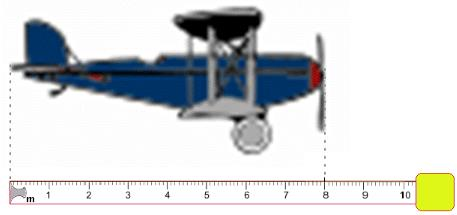Solution:

The unit of measurement is m.
So the plane is 8 m long.

QUESTION: 2

### The ruler shows that the length of the pencil is 12. What is the unit of this length?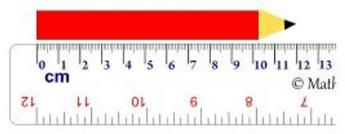Solution:

The unit of measurement is cm.
So the pencil is 12 cm long.

QUESTION: 3

### Imagine you are given the following four measuring instruments: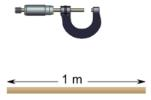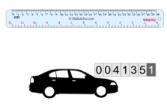A. A micrometer, which is a special instrument used by engineers to accurately measure to the nearest millimeter or even more precisely. B. A 30 cm ruler, which is the ruler you usually use in school. C. A stick exactly one meter long. D. An odometer, which is an instrument used in a car to measure distances in kilometers. Which instrument would you use to roughly measure the length of your classroom?

Solution:

You would not be looking for an accurate answer, so you would use a stick exactly one meter long, and see how many times you need to use the stick.

QUESTION: 4

Two traffic signs mark the bridge in my village: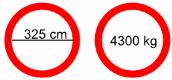These signs indicate the maximum vehicle width and the maximum vehicle weight allowed on the bridge.

Which one of the following trucks is allowed to cross that bridge?

Solution:

'Maximum' means the greatest that is allowed.
In case A, the weight (4400 kg) is too much.
In case B, the weight (4400 kg) is too much.
In case C, the width (330 cm) is too much.
In case D, the width (325 cm) is on the maximum and the weight (4250 kg) is below the maximum. So D is allowed to cross the bridge.

QUESTION: 5

Imagine you are given the following four measuring instruments: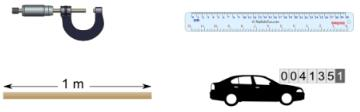A. A micrometer, which is a special instrument used by engineers to accurately measure to the nearest millimeter or even more precisely.
B. A 30 cm ruler, which is the ruler you usually use in school.
C. A stick exactly one meter long.
D. An odometer, which is an instrument used in a car to measure distances in kilometers.

Which instrument would a telephone engineer use to measure the size of a small component?

Solution:

He would have to measure accurately, so he would use a micrometer.

QUESTION: 6

This pencil has length 120. What is the unit of this length?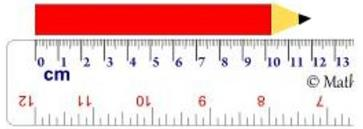Solution:

The pencil is 12 cm long.
12 cm = 120 mm
So, the unit of measurement is mm.

QUESTION: 7

Imagine you are given the following four measuring instruments: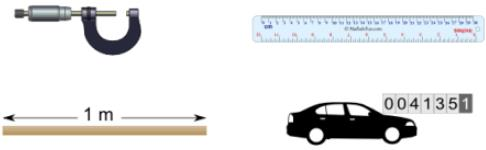A. A micrometer, which is a special instrument used by engineers to accurately measure to the nearest millimeter or even more precisely.
B. A 30 cm ruler, which is the ruler you usually use in school.
C. A stick exactly one meter long.
D. An odometer, which is an instrument used in a car to measure distances in kilometers.

Which instrument would a taxi driver in a large city use to measure the distance from the city center to the airport?

Solution:

He would  measure the distance using the taxi's odometer.

QUESTION: 8

The measuring tape shows that the length of the plane is 800. What is the unit of this length?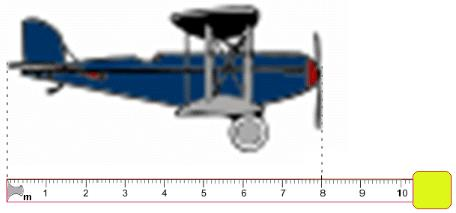Solution:

The plane is 8 m long.
8 m = 800 cm
So the unit of measurement is cm.

QUESTION: 9

Imagine you are given the following four measuring instruments:A. A micrometer, which is a special instrument used by engineers to accurately measure to the nearest millimeter or even more precisely.
B. A 30 cm ruler, which is the ruler you usually use in school.
C. A stick exactly one meter long.
D. An odometer, which is an instrument used in a car to measure distances in kilometers.

Which instrument would you use to measure the length of your index finger?

Solution:

You would use an ordinary ruler to measure the length of your index finger to the nearest millimeter.

QUESTION: 10

Which unit would you most likely use to measure the width of the cover of a paperback book?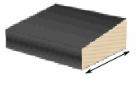Solution:

The width of the cover of a paperback book is usually about 11 centimeters, so you would use centimeters.

QUESTION: 11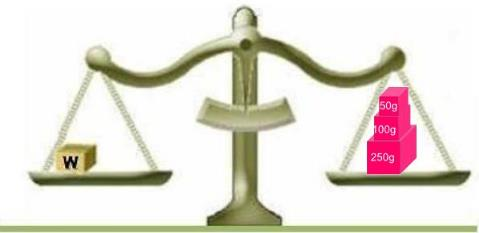The scales are balanced.
What is the value of the weight W in grams?

Solution:

W = 50g + 100 g + 250 g = 400 g

QUESTION: 12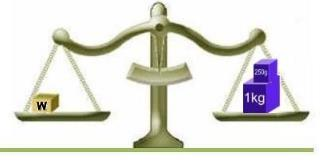The scales are balanced.
What is the value of the weight W in grams?

Solution:

W = 1 kg + 250 g = 1000 g + 250 g = 1250 g

QUESTION: 13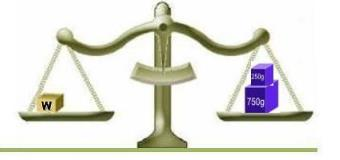The scales are balanced.
What is the value of the weight W in grams?

Solution:

W = 250g + 750 g = 1000 g

QUESTION: 14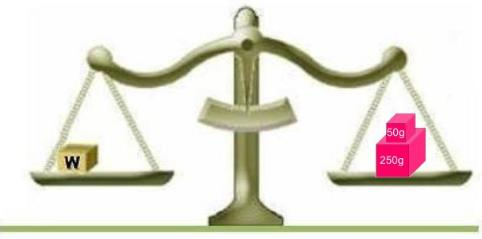The scales are balanced.
What is the value of the weight W in grams?

Solution:

W = 50g + 250 g = 300 g

QUESTION: 15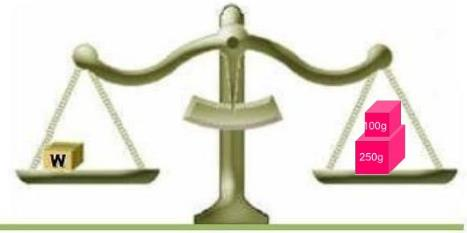The scales are balanced.
What is the value of the weight W in grams?

Solution:

W = 100g + 250 g = 350 g

QUESTION: 16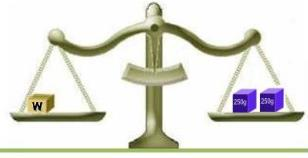The scales are balanced.
What is value of the weight W in grams?

Solution:

W = 250g + 250 g = 500 g

QUESTION: 17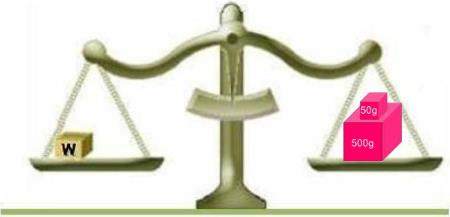The scales are balanced.
What is the value of the weight W in grams?

Solution:

W = 50g + 500 g = 550 g

QUESTION: 18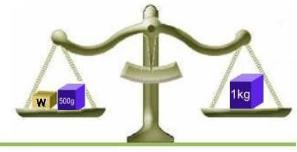The scales are balanced.
What is value of the weight W in grams?

Solution:

W and 500 g together balance with 1 kg = 1000 g.
So W must be 1000g − 500 g = 500g.

QUESTION: 19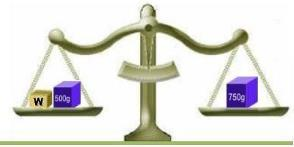The scales are balanced.
What is value of the weight W in grams?

Solution:

W and 500 g together balance with 750g
So W must be 750g − 500 g = 250g.

QUESTION: 20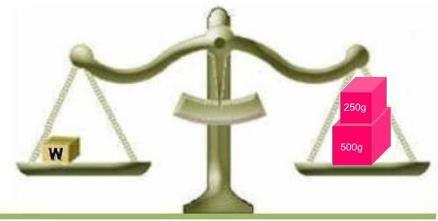The scales are balanced.
What is the value of the weight W in grams?

Solution:

W = 250g + 500 g = 750 g